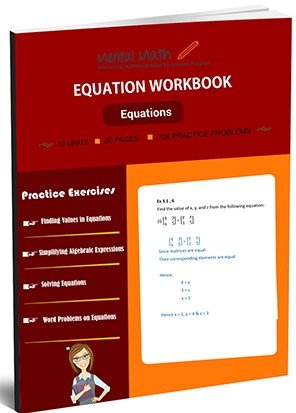20,864

# Equation Worksheets\$7.00

An open statement in which two expressions are equal is called an equation. Students start this section finding values in the equations. They evaluate algebraic expressions and simplify them to be preparing to solve the equations. After the discussion of properties of equations, students practice solving simple equations, equation with parentheses, variables on two sides of the equations, and word problems of equations related to time and percent.

Worksheet # Topics/Curriculum # of Pages Sample Add to cart
Unit-1 Finding Values in Equations 8 pages\$1.00

Unit-2 Evaluating Algebraic Expressions 8 pages\$1.00

Unit-3 Adding and Subtracting Algebraic Expressions 8 pages\$1.00

Unit-4 More Problems on Adding and Subtracting Algebraic Expressions 8 pages\$1.00

Unit-5 Simplifying Algebraic Expressions 8 pages\$1.00

Unit-6 Solving Equations 8 pages\$1.00

Unit-7 More Problems on Solving Equations 8 pages\$1.00

Unit-8 Solving Equations with Parentheses 8 pages\$1.00

Unit-9 Advanced Problems on Solving Equations 8 pages\$1.00

Unit-10 Word Problems on Equations 8 pages\$1.00

## Choose From Over 15 Worksheets That Best Suit Your Child’s Skill Level and Unique Needs

You can purchase our high quality math worksheets separately, or purchase an entire book and save 40%!

### Equation Worksheets

Evaluate Algebraic Expressions and Solve Equations

See all units

### Integers Worksheets

Four Operations on integers and their Simplification

See all units

### Decimals Worksheets

Decimal Concept and Four Operations on Decimals

See all units

### Fractions Worksheets

Fraction Concepts and Four Operations on Fractions

See all units

### Division Worksheets

Dividing up to 4 or more digit Numbers by 2 or 3-digit Numbers

See all units

### Multiplication Worksheets

Multiplication Table of 2 through 10 and Multiplication by Thousands

See all units

### Subtraction Worksheets

Subtracting 4 to 1-digit Numbers form 4 digit Numbers

See all units